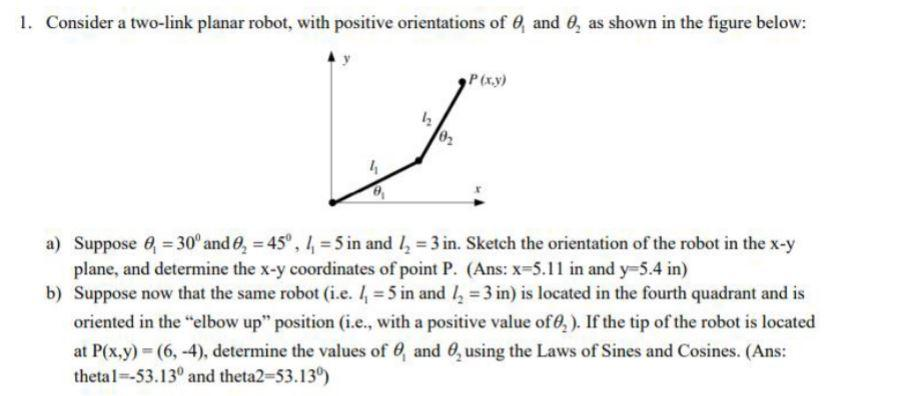# Question Solved1 Answer1. Consider a two-link planar robot, with positive orientations of 0, and 0, as shown in the figure below: P(x,y) h 02 a) Suppose , = 30° and , = 45°, 1= 5 in and l= 3 in. Sketch the orientation of the robot in the x-y plane, and determine the x-y coordinates of point P. (Ans: x=5.11 in and y=5.4 in) b) Suppose now that the same robot (i.e. 11 = 5 in and ly = 3 in) is located in the fourth quadrant and is oriented in the elbow up" position (i.e., with a positive value of 0,). If the tip of the robot is located at P(x,y) = (6,-4), determine the values of , and 0, using the Laws of Sines and Cosines. (Ans: thetal --53.130 and theta2-53.139)Transcribed Image Text: 1. Consider a two-link planar robot, with positive orientations of 0, and 0, as shown in the figure below: P(x,y) h 02 a) Suppose , = 30° and , = 45°, 1= 5 in and l= 3 in. Sketch the orientation of the robot in the x-y plane, and determine the x-y coordinates of point P. (Ans: x=5.11 in and y=5.4 in) b) Suppose now that the same robot (i.e. 11 = 5 in and ly = 3 in) is located in the fourth quadrant and is oriented in the elbow up" position (i.e., with a positive value of 0,). If the tip of the robot is located at P(x,y) = (6,-4), determine the values of , and 0, using the Laws of Sines and Cosines. (Ans: thetal --53.130 and theta2-53.139)
More
Transcribed Image Text: 1. Consider a two-link planar robot, with positive orientations of 0, and 0, as shown in the figure below: P(x,y) h 02 a) Suppose , = 30° and , = 45°, 1= 5 in and l= 3 in. Sketch the orientation of the robot in the x-y plane, and determine the x-y coordinates of point P. (Ans: x=5.11 in and y=5.4 in) b) Suppose now that the same robot (i.e. 11 = 5 in and ly = 3 in) is located in the fourth quadrant and is oriented in the elbow up" position (i.e., with a positive value of 0,). If the tip of the robot is located at P(x,y) = (6,-4), determine the values of , and 0, using the Laws of Sines and Cosines. (Ans: thetal --53.130 and theta2-53.139)